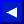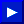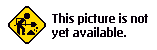as a special way of counting. Here's a close-up view of the first eight pixels in the top left hand corner of the sprite:#### STEP 4:

Add up the numbers of the SOLID pixels. This first group of eight pixels is completely solid, so the total number is 255.

#### STEP 5:

Enter that number as the FIRST DATA STATEMENT in line 100 of the Spritemaking Program below. Enter 255 for the second and third groups of eight.

#### STEP 6:

Look at the FIRST EIGHT PIXELS IN THE SECOND ROW of the sprite. Add up the values of the solid pixels. Since only one of these pixels is solid, the total value is 128. Enter this as the first DATA number in line 101.#### STEP 7:

Add up the values of the next group of eight pixels (which is 0 because they're all BLANK) and enter in line 101. Now move to the next group of pixels and repeat the process for each GROUP OF EIGHT PIXELS (there are 3 groups across each row, and 21 rows). This will give you a total of 63 numbers. Each number represents ONE group of 8 pixels, and 63 groups of eight equals 504 total individual pixels. Perhaps a better way of looking at the program is like this... each line in the program represents ONE ROW in the sprite. Each of the 3 numbers in each row represents ONE GROUP OF EIGHT PIXELS. And each number tells the computer which pixels to make SOLID and which pixels to leave blank.This page has been created by Sami Rautiainen. Read the small print. Last updated May 12, 2002.